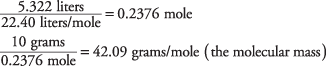## Ideal Gas Equation

The relations known as Boyle's law, Charles' law, and Avogadro's law can be combined into an exceedingly useful formula called the Ideal Gas Equation,

PV = nRT

where R denotes the gas constant:The temperature is, as always in gas equations, measured in Kelvin.

This formula is strictly valid only for ideal gases—those in which the molecules are far enough apart so that intermolecular forces can be neglected. At high pressures, such forces cause significant departure from the Ideal Gas Equation, and more complicated equations have been devised to treat such cases. The Ideal Gas Equation, however, gives useful results for most gases at pressures less than 100 atmospheres.This is the value stated in the carbon dioxide reaction; you were asked to memorize that 1 mole of any gas occupies 22.4 liters at STP.

You should be able to use the Ideal Gas Equation to determine any one of the four quantities—pressure, volume, moles, or temperature—if you are given values for the other three.

One important application is to deduce the molecular mass and formula for a gas. Assume that you know that the hydrocarbon propylene is, by mass, 85.6% carbon and 14.4% hydrogen. Then the atomic ratios of the compound areTherefore, the propylene molecule is some integral multiple of CH 2: It can be CH 2 or C 2H 4 or C 3H 6 or a yet larger molecule. Measuring the volume of 10 grams of propylene at STP yields 5.322 liters, which you can use to calculate its molecular mass.Because the atomic masses of 1 CH 2 unit added together is 14.03, the molecule contains three such units. Consequently, the molecular formula for propylene is C 3H 6.

• What is the volume occupied by 1 kilogram of carbon monoxide at 700°C and 0.1 atm?
• The ozone molecule contains only oxygen atoms. Determine the molecular formula of ozone given that 2.3 grams occupies 1,073 milliliters at standard temperature and pressure.